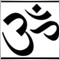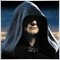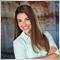# Kelly Criterion...670

Hi,

Have been testing Kelly MM, the results have been mixed but overall there seems to be more profit and less DD and losses.

But... I've sampled less than all trades, typically the 6 - 30 last trades were used in the Kelly calculations.

Hard limit was set at 0.3 x Kelly, although I've seen the EA trade 'well' and survive with up to 60% risk pr. trade (!).

Now... with a limited number of Kelly-samples (as mentioned above) the Kelly will tend to saturate, this always happen on

long loosing or winning streaks. Eg. if I use 6 Kelly-samples and those trades were all losers, Kelly wants to trade with 0% risk.

This is something I can agree to. But if those trades were all winners Kelly would want to trade with 100% risk... very profitable

but in the real world obviously this is all wet and all wrong.

Using a Kelly factor of 0.2 or thereabout goes a long way to approach reality (if you have the stomach to trade @ 20% risk)

but I still feel there is something missing... as if the Kelly is incomplete or unfinished...

Any views and suggestions ?5299

My personal interpretation of Kelly is that it's best applied to mathematical systems. Trading systems which are not purely mathematical would not benefit from Kelly. A mathematical system is one where you can calculate your edge based on math. Using a simulator can then help you confirm the math. When I toke up Black-Jack, the conventional wisdom was that you'd need something on the scale of a Million-Independent Events/Trials to get a good handle on your edge. Not to mention Blackjack is a game which can be Proven Mathematically.

If somehow the statistics from the Tests will stay the same in the future (strongly doubt it), then bingo, even I can't argue with Kelly there. So yea, you're making a mistake by using Kelly on anything less then a Huge-Number-Of-Trades, you need the number of trades to be so big that the probability of Luck/Curve-Fit is un-likely. I'll go with Blackjack's approach here and say a couple million non-series (not---grid/martingale/pyramid/partial-close etc) trades. That or find a purely mathematical approach and use your Kelly with that.

If I was to employ Kelly, I'd use 25%-50% the Kelly recommendation based on my All-Combined Years/Symbol back-test. I'll not use it as some form of Recent Trades (System in the Zone) indicator. <---- You can try looking at WHRoeder's code and searching for Trade Encourage Factor ... But all these thing are Theoretical. But even with 100-years of trades and employing Kelly, I wouldn't dare fool myself into thinking A) the account cannot go broke. and B) I'll always be able to take small (or large) consistent profits from the market. My personal psychology on Forex is that 1) I'm here to make money and 2) If I'm not willing to lose it, it wouldn't be in the Account.1091

Hi,

Have been testing Kelly MM, the results have been mixed but overall there seems to be more profit and less DD and losses.

Using a Kelly factor of 0.2 or thereabout goes a long way to approach reality (if you have the stomach to trade @ 20% risk)

but I still feel there is something missing... as if the Kelly is incomplete or unfinished...

Any views and suggestions ?

You could try googling Optimal F (by Ralph Vince). Also google "ralph vince experiment" I think this is strongly related to Kelly and fractional Kelly but more focused on trading.

What's interesting about Optimal F (& Kelly) is that it will not make an unworkable system workable, but it can make a workable system unworkable (=unprofitable), specifically if you reinvest too much compared to the profit factor of the system.

EDIT: for clarity. Optimal f is at the peak value of a profit curve. Either side of it gives a less optimal result. Re-investing too much (ie going past the optimal point) can take a winning system into a losing system. If your estimate of profit factor is too high then you will calculate too high a trade size and the system will become unprofitable. Arguably you are now not using Optimal F. The point is that in the real world you have to calculate based on the existing data.5299

Folks in the trading world like to complain about Kelly or Optimum-f being too risky. Folks in the trading world also like to think 30-trades are meaningful in terms of calculation Kelly. Those are all very bad assumptions which leads to the criticism of Kelly or Optimum-f.

Folks like to think their back-tested systems are Static / Black-Boxes / Holy-Grails. Kelly itself can-never make a profitable system un-profitable unless the system is lying about its statistics in the first place.

Profit-factor is a nice matrix for using as fractional-size. But what happens when one uses profit-factor on the last 6-to-30 trades and get all winners. Profit-factor will tell you to risk 100%. This should make sense as if there's no chance of one losing then one should risk 100%. Now should one lose, one cannot turn around and say Profit-factor made my profitable system un-profitable. In the same way one cannot look at Kelly and say the same :).5299

Decided to add the codes here (outside of the Mathematics thread) for someone searching for Kelly or Optimal-f stuff. There's also this link which explains different types of MM's. There's another one which could be easily added to the codes below. The code below is-not in lot-size as the function name would suggest. Rather its the Full-Kelly fraction of risk-per-trade.

Ps> Just by looking at the math, one can see that Kelly works in Units (Win vs Loss). Where as Optimal-f works in \$\$\$ (Amount-Won vs Amount Loss). That to me seems the be the biggest difference between the two.

```//~~~~~~~~~~~~~~~~~~~~~~~~~~~~~~~~~~~~~~~~~~~~~~~~~~~~~~~~~~~~~~~~~~~~~~~~~~~~~~~~~~~~~~~~
//~~~~~~~~~~~~~~~~~~~~~~~~~~~~~~~~~~~~~~~~~~~~~~~~~~~~~~~~~~~~~~~~~~~~~~~~~~~~~~~~~~~~~~~~
double Lot_Size(int Switch){
static int Saved_His_Total;
int His_Total=OrdersHistoryTotal();
if(His_Total==0){return(0.1);}
if(Saved_His_Total != His_Total){
Saved_His_Total=His_Total;
for(int i=His_Total; i>=0; i--){
if(OrderSelect(i, SELECT_BY_POS, MODE_HISTORY)
&& OrderMagicNumber()==Magic
&& OrderSymbol()==Symbol()
){
static int Buy_Wins; static int Sel_Wins;
static int Buy_Loss; static int Sel_Loss;
static double Sel_Profit, Sel_Losses;
if(OrderType()==OP_SELL && OrderProfit()>0){
Sel_Wins++; Sel_Profit+=OrderProfit();}
if(OrderType()==OP_SELL && OrderProfit()<=0){
Sel_Loss++; Sel_Losses+=OrderProfit();} break;
}   }
double Win_Total = Buy_Wins + Sel_Wins;
double Loss_Total = Buy_Loss + Sel_Loss;
double Profit_Total = Buy_Profit + Sel_Profit;
double Losses_Total = Buy_Losses + Sel_Losses;
if(Win_Total !=0){double Avg_Profit = Profit_Total / Win_Total;}
if(Loss_Total!=0){double Avg_Losses = Losses_Total / Loss_Total;}
if(Avg_Losses !=0){double P2L_Ratio = Avg_Profit / Avg_Losses;}
if(Avg_Losses !=0){double Kd=Avg_Profit/Avg_Losses;}
//~~~~~~~~~~~~~~~~~~~~~
if(W2L_Ratio !=0){double RvRoulette=(0.47/W2L_Ratio)*0.1;}
//~~~~~~~~~~~~~~~~~~~~~
if(Kd !=0){double Kelly=(W2L_Ratio-L2W_Ratio)/(Avg_Profit/Avg_Losses);}
if(P2L_Ratio!=0){double Optimal_f=((P2L_Ratio + 1)*W2L_Ratio-1)/P2L_Ratio;}
//~~~~~~~~~~~~~~~~~~~~~
if(Switch=='R'){return(RvRoulette);}
if(Switch=='K'){return(Kelly);}
if(Switch=='F'){return(Optimal_f);}
//~~~~~~~~~~~~~~~~~~~~~
}
}
//~~~~~~~~~~~~~~~~~~~~~~~~~~~~~~~~~~~~~~~~~~~~~~~~~~~~~~~~~~~~~~~~~~~~~~~~~~~~~~~~~~~~~~~~
//~~~~~~~~~~~~~~~~~~~~~~~~~~~~~~~~~~~~~~~~~~~~~~~~~~~~~~~~~~~~~~~~~~~~~~~~~~~~~~~~~~~~~~~~```1161

Kelly is used more for risk/reward and accuracy.